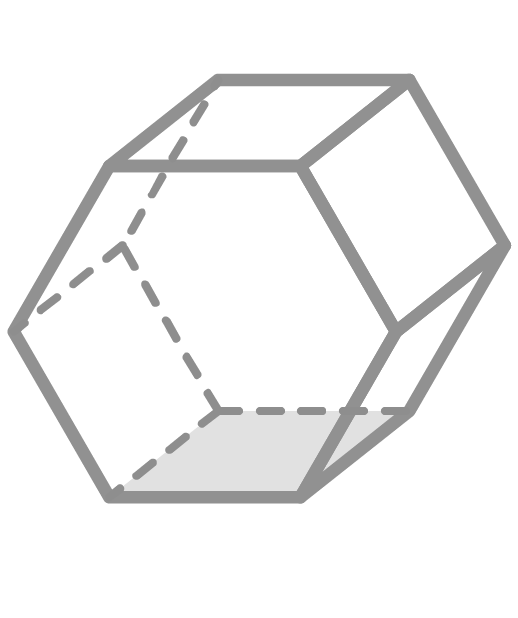Chapter OverviewMaths

Types of numbers

Number calculations

Fractions, decimals and percentages

Algebraic manipulation

Formulae and equations

Straight line graphs

Other graphs

Ratio

Proportion

Rates of change

Shapes

Properties of shapes

Measures

Lines and angles

Drawing shapes

Trigonometry

Probability

Statistics

Maths

# Nets and surface area0%

Summary

# Nets and surface area

## ​​In a nutshell

Finding the surface area of a 3D shape is much easier to approach by using nets. A net of a 3D shape is a 2D representation of the faces of the 3D shape.

## Nets

### Definition

A net is a 3D shape that has been unfolded and laid out flat. There is often more than one net for a particular shape. Here are some examples of nets.

 ​​SHAPE NET​## Finding surface area using nets

Finding the surface area of a 3D shape is the same as finding the total area of the net of the shape. A net is usually a compound shape, so you can find the area of the net by working out the areas of the individual shapes.

##### Example 1

A cuboid has base, width and height to be $4cm, 7cm$ and $5cm$ respectively. Work out the surface area of the cuboid.

First, sketch the net of the cuboid. The cuboid is made up of six rectangles that come in three pairs, so the net will look like this:

Now, work out the area of the net:

The net has two rectangles with side lengths $4cm$ and $7cm$. So, their combined area is $2\times4\times7=56cm^2$.

The net has two rectangles with side lengths $7cm$ and $5cm$. So, their combined area is $2\times7\times5=70cm^2$.

The net has two rectangles with side lengths $4cm$ and $5cm$. So, their combined area is $2\times4\times5=40cm^2$.

Then, add up all the individual areas to give the total area of the net - and hence the surface area:

$56+70+40=166$​​

The surface area of the cuboid is $\underline{ 166 \ cm^2}$.

## Cylinders

The net of a cylinder consists of two circles and a rectangle.While it is possible to use the net to work out the surface area, it is easier to just memorise the formula in this case.

$\text{Surface area of a cylinder}=2\pi r^2+2\pi rh$

Where $r$ is the radius, and $h$ is the height of the cylinder.

##### Example 2

What is the exact surface area of a cylinder with a radius of $7cm$ and a height of $1cm$?

Substitute $r=7,h=1$ into the formula:

\begin{aligned}\text{Surface area of a cylinder}&=2\pi r^2+2\pi rh\\&=2\pi (7)^2+2\pi (7)(1)\\&=2\pi (49) + 2\pi (7)\\&= 98\pi + 14\pi\\&=112\pi \end{aligned}​​

The question is asking for the exact surface area, so leave the answer in terms of $\pi$.

The surface area of the cylinder is $\underline{112\pi \ cm^2}$.

FAQs

• Question: What is the formula for the surface area of a cylinder?

Answer: The surface area of a cylinder is given to be SA=2πr^2+2πrh.

• Question: How do you work out the surface area of a shape using its net?

Answer: First, sketch the net. Then, work out the area of the net by working out the area of each individual shape in the net.

• Question: What is a net?

Answer: A net is a 3D shape that has been unfolded and laid out flat.

Theory

Exercises